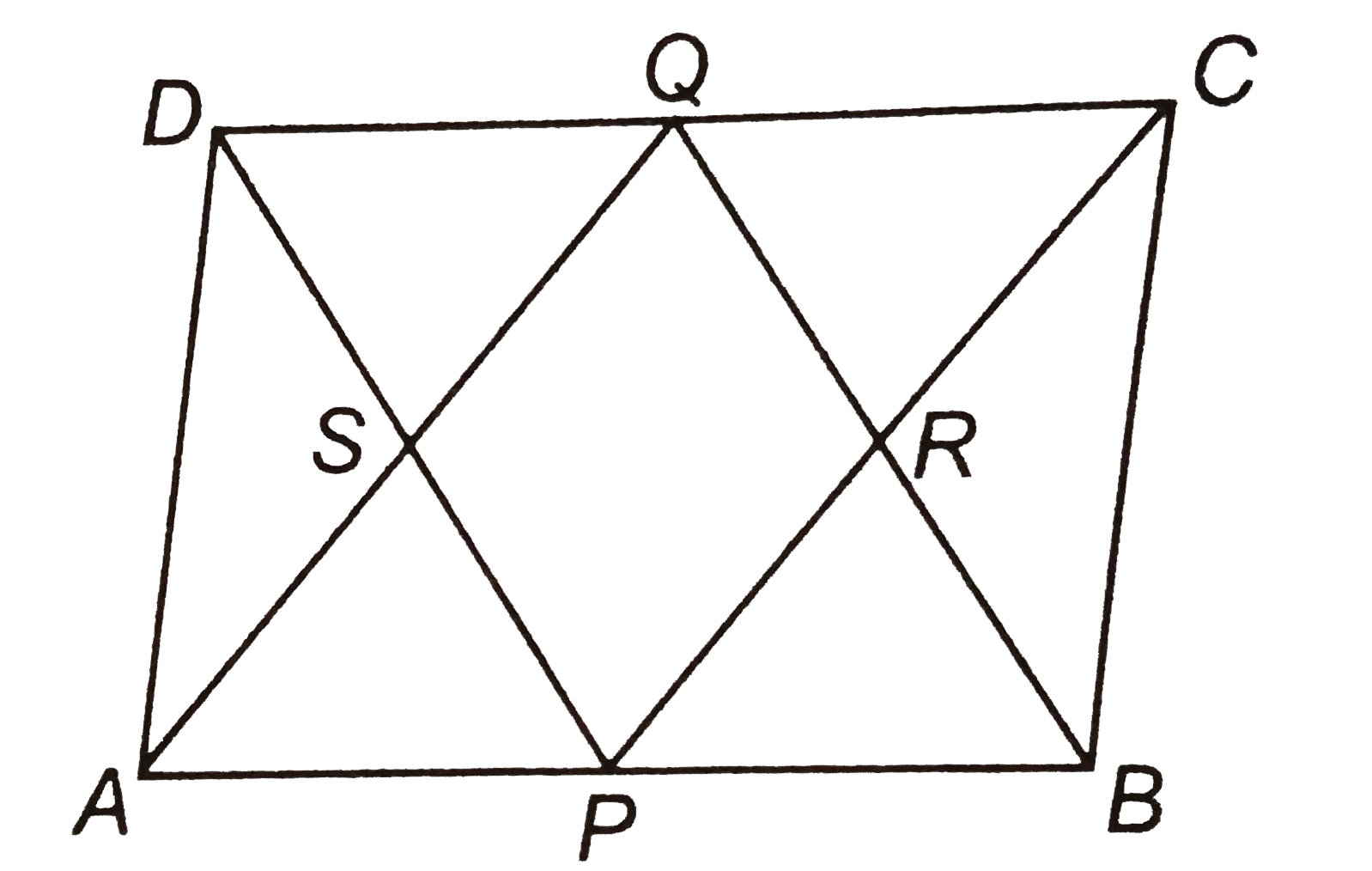# ABCD is a parallelogram in which P and Q are mid-points of opposite sides AB and CD (see Fig. 8.18). If AQ intersects DP at S and BQ intersects CP at

92 views

closed
ABCD is a parallelogram in which P and Q are mid-points of opposite sides AB and CD (see Fig. 8.18). If AQ intersects DP at S and BQ intersects CP at R, show that: (i) APCQ is a parallelogram. (ii) DPBQ is a parallelogram. (iii) PSQR is a parall

by (97.1k points)
selected by

Given In a parallelogram ABCD, P and Q are the mid-points of AB and CD, respectively.
To show PRQS is a parallelogram.
Proof Since, ABCD is a parallelogram.
" "AB||CD" "
rArr" "AP||QC
Also, " "AB=DC" "(1)/(2)AB=(1)/(2)DC" " [dividing both sides by 2]
rArr" "AP=QC" " [ since, P and Q are the mid-points of AB and DC]
Now, " "AP||QC and AP=QC
Thus APCQ is a parallelogram.
therefore" "AQ||PC or SQ||PR" "...(i)
Again, " "AB||DC or BP||DQ
Also, " "AB=DC rArr(1)/(2)AB=(1)/(2)DC" " [dividing both sides by 2]
rArr" "BP=QD [since, P and Q are the mid-points of AB anc DC]
Now, " "BP||QD and BP=QD
So, BPDQ is a parallelogram.
therefore" "PD||BQ or PS||QS" "...(iii)
From Eqs. (i) and (ii), " "SQ||RQ and PS||QR
So, PRQS is a parallelogram. " " Hence proved.